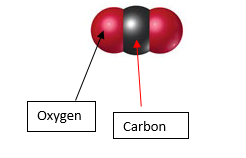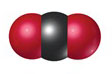# Problem: Calculate the percentage of carbon by mass in each of the compounds represented by the following models. (Carbon atoms are black spheres, hydrogen are grey, and oxygen are red.)

###### FREE Expert Solution

The percentage of carbon by mass in the compound shown can be found as follows:

Step 1: Count the number of atoms represented in the model.###### Problem Details

Calculate the percentage of carbon by mass in each of the compounds represented by the following models. (Carbon atoms are black spheres, hydrogen are grey, and oxygen are red.)